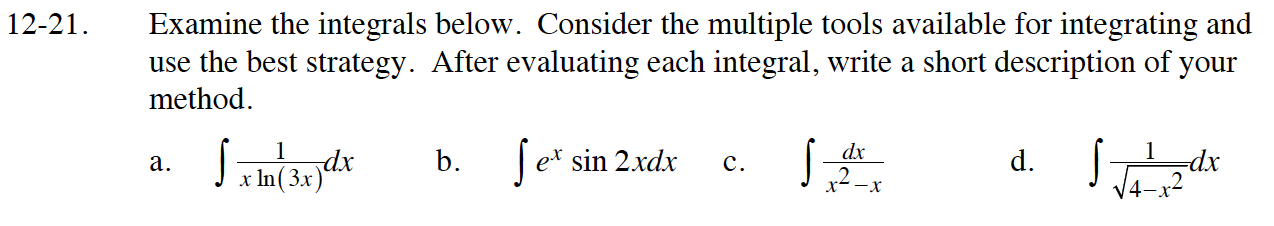### Home > CALC > Chapter Ch12 > Lesson 12.1.2 > Problem12-21

12-21.Use substitution. Let u = ln(3x).

Use integration by parts. Let f = sin(2x) and dg = exdx.
Then df = 2cos(2x)dx and g = ex.

$=e^x\sin(2x)-2\int e^x\cos(2x)dx$

Use integration by parts on the integral in Step 2.
Let f = cos(2x) and dg = exdx.
Then df = –2sin(2x)dx and g = ex.

Use partial fraction decomposition.

$\frac{1}{x^2-x}=\frac{A}{x}+\frac{B}{x-1}$

$\sqrt{4-x^2}=\sqrt{4(1-\frac{x^2}{4})}$

$=2\sqrt{1-\Big(\frac{x}{2}\Big)^2}$

Rewrite the denominator of the integrand using Steps 1 and 2.
You should recognize the integrand as a form of the derivative of a special function.

Use algebra to get the original integral expression by itself.